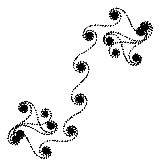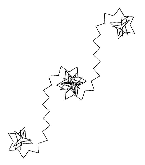Mathematical and Physical Journal
for High Schools
Issued by the MATFUND Foundation
 Already signed up? New to KöMaL?

#Problem I. 147. (December 2006)

I. 147. The following algorithm generates some spiral-like figures. Consider a horizontal line segment, then, in every step, the starting point of the next, nth line segment and the endpoint of the previous one is joined in such a way that these segments encompass an angle of bn, where numbers bn are members of an arithmetic progression bn-bn-1=d with constant d. In the construction each segment has the same length.

Prepare your program to display these spirals. The input consists of the number d (real number) and the common length L (positive real number) of the segments. A message should notify the user when all possible line segments have been drawn (that is, when repetition occurs). See the example.d=24,01   L=1,5d=177   L=10

The source code of the program (i147.pas, i147.cpp, ...) should be submitted.

(10 pont)

Deadline expired on January 15, 2007.

Sorry, the solution is available only in Hungarian. Google translation

Az alábbi file tartalmazza a feladat mintamegoldását.

### Statistics:

 16 students sent a solution. 10 points: Biró János, Czigler András, Danka Miklós András, Szoldatics András, Vincze János. 9 points: Gilián Zoltán. 8 points: 4 students. 6 points: 1 student. 5 points: 2 students. 4 points: 1 student. 2 points: 2 students.

Problems in Information Technology of KöMaL, December 2006